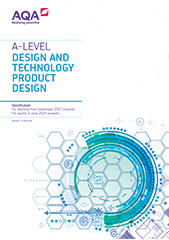# Appendix: Links to maths and science

All A-level specifications in design and technology must require students to demonstrate their application of knowledge, understanding and skills of maths and science in both theoretical and practical ways. Design and technology uses maths and science to support decisions made in the processes of designing and making.

## Maths

Ref

Maths skills requirement

Potential applications: product design

a

Confident use of number and percentages

Calculation of quantities of materials, costs and sizes

b

Use of ratios

Scaling drawings

c

Calculation of surface areas and/or volumes

Determining quantities of materials

d

Use of trigonometry

Calculation of sides and angles as part of product design

e

Construction, use and/or analysis of graphs and charts

Representation of data used to inform design decisions and evaluation of outcomes.

Presentation of market data, user preferences, outcomes of market research

f

Use of coordinates and geometry

Use of datum points and geometry when setting out design drawings

g

Use of statistics and probability as a measure of likelihood

Interpret statistical analyses to determine user needs and preferences.

Use data related to human scale and proportion to determine product scale and dimensions

## Science

Ref

Scientific knowledge and skills

Potential applications: product design

a

Describe the conditions which cause degradation

Ensure products are designed to take account of potential corrosion due to environmental factors

b

Know the physical properties of materials and explain how these are related to their uses

Understand the appropriate use of materials, including glass and ceramics, polymers, composites, woods, and metals, based on their physical properties